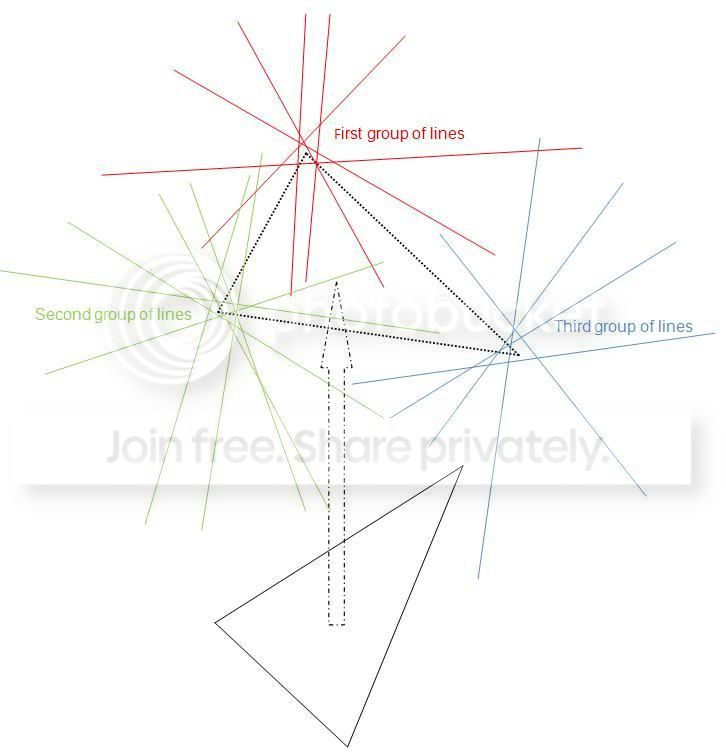# Rigid-body matching algorithm and clustering algorithm with groups of lines in 3D

I've been struggling with this problem for weeks, and couldn't find an appropriate algorithm to solve it. Could you guys please give me some advices or suggestions in addressing this question. Or if you know anyone who could answer this question, please send it to them. I really need the solution as I have been trying to figure it out for quite long time :| Many thanks.

As can be seen in the picture below, I have more than 200 lines in 3D. They consist of 3 different groups of lines, and the common property of the lines in each group is that they pass close to the same point. However, in this problem, we don't know which line belongs to which group. What is given are only the 3D coordinates (x,y,z) of the 2 ends of all the lines.

My job is to find an algorithm to match/fit/register a triangle (a rigid body) to those lines such that the sum of the sum of distances between 3 vertexes of the triangle and their corresponding lines is minimized. In some cases, one vertex may be the closest point in a group, but the other vertexes would be dragged further away from their corresponding lines (because they are in a rigid body), thus this sum will not be minimal. What I need is the best positions of the 3 vertexes (the best fit of the triangle) to minimize the global sum.

In conclusion, given more than 200 sets of (x1,y1,z1,x2,y2,z2) (which are coordinates of 2 ends of a line), I need to find the best positions of 3 vertexes of a triangle to fit in those lines (given the relative distance between the 3 vertexes).

Without the rigid-body condition, I have successfully determined the positions of the 3 independent points using the Expectation-Maximization (EM) clustering algorithm. However, sometimes 2 or 3 points are assigned to the same position. Then, I realized I had forgotten the "rigid-body" condition. With this added condition, this error will not occur. In the EM algorithm, I can derive the formula for each repositioning step for each point. But when it comes to a rigid-body, I don't know how to figure out the translation and rotation matrix to fit the triangle to the lines.

Thank you for reading this post. Your help would be greatly appreciated.• I don't think the "cluster-algebras" or "line-bundles" tags fit. – Douglas Zare Aug 30 '12 at 10:21
• Thank you for the correction. I have removed them in the tags list. – Duc Trung Aug 30 '12 at 11:05
• What do you mean by saying that " sometimes 2 or 3 points are assigned to the same position."? – Felix Goldberg Aug 30 '12 at 11:10
• I think you could determine reasonable approximate locations for the three "meeting points" using, say, RANSAC. Then it'd be relatively simple, I think, to deform optimally the triangle generated by them into one congruent to the given one. But you probably know all that. So what am I missing? – Felix Goldberg Aug 30 '12 at 11:13
• @Felix: in some cases, when the number of lines in one group is too small compared to that of the other groups, (or due to noise), 2 points converge to the same position. At the end of the iteration, I obtain 3 points but two or all of them are actually identical. That's why I said "sometimes 2 or 3 points are assigned to the same position". But this is for the case of independent points. For the case when rigid-body condition is added, I think this error will not occur. (Sorry about my poor English. I've tried to explain it as clearly as possible. Hope that makes sense) – Duc Trung Aug 30 '12 at 12:47